# 8.3.2 Bubble Sort | Sorting in C++ | Guaranteed Placement CourseSame notes as Lecture 9.3.1

## 23 thoughts on “8.3.2 Bubble Sort | Sorting in C++ | Guaranteed Placement Course”

1.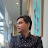sourabh singh says:

can anyone explain this
arrey- 31 11 27 2 19
it has 5 elements and according to this video this array should be sorted in 4 steps but in this case, it gets sorted in only 3 steps.
if this is happening then the compiler will iterate this one more time and which will increase compilation time.
can anyone explain this
Thank You.

2.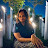C_57 _Muskan Singh says:

🦋💚☘️🍁

3.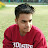Mohit Aggarwal says:

every college guy knows that urvi's gonna face a lot of simping after recording this in sucha lovely voice XD

4.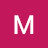Moreshwar Mujbaile says:

3:55 pe n-1 ke jagah n-0 (and so on) hona chaiye shyd
IDK i am not sure about that ??
Can someone explain plz…

5.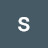suraj Sharma says:

45 deslike walo ke liye suar hmesa kichad m hi khus rhte hai🖕🖕

6.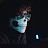Musheer Jamadar says:

Bgm koi bata do

7.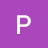Prabhdeep Singh says:

love u guys

8.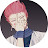Hisoka Morrow says:

apart from using 'while + for' loop, one can also use double-for loops:

for(int i=0;i<n-1;i++){

for(int j=0;j<(n-i);j++){

if(arr[j]>arr[j+1]){

int temp=arr[j];

arr[j]=arr[j+1];

arr[j+1]=temp;

}

}

}

9.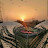193 Anurag T says:

Please make a video on how to make a floating donut in code

10.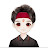krish Sharma says:

Thanks ☺️

11.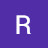Rishil Trivedi says:

we can execute this code by using for loop also.

#include<iostream>
using namespace std;
int main(){
int n;
cin>>n;

int arr[n];
for(int i=0;i<n;i++){
cin>>arr[i];
}

for(int counter=1;counter<n;counter++){
for(int i=0;i<n-counter;i++){
if(arr[i]>arr[i+1]){
int temp=arr[i+1];
arr[i+1]=arr[i];
arr[i]=temp;
}
}
}
for(int i=0;i<n;i++){
cout<<arr[i]<<endl;

}
}

12.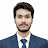Dipak says:

int arr[n] is showing an error. It says the array should be declared.

13.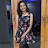Prajna Gupta says:

This is awesome! Very concise and informative😍

14.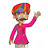gujju carry says:

Hi everyone what's up….at 0:01👌👌👌

15.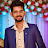0038 Uttam Karmakar says:

I don't think anyone can explain the bubble concept so easily than how our Didi teaches us 😍❤️
* The condition inside while loop should be counter<n-1

16.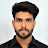ABHINAV SHARMA says:

Another way to write the same code using nested for loops:
#include<iostream>

using namespace std;

int main()

{

int c;

cin>>c;

int arr[c];

for(int i=0;i<c;i++)

{

cin>>arr[i];

}

for(int i=c-1;i>=0;i–)

{

for(int j=0;j<i;j++)

{

if(arr[j+1]<arr[j])

{

int temp=arr[j];

arr[j]=arr[j+1];

arr[j+1]=temp;

}

}

}

for(int i=0;i<c;i++)

{

cout<<arr[i]<<" ";

}

cout<<endl;

}

17.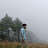SHUBHAM BARTWAL says:

how will you achieve 0(n) complexity in the best case using this method?

18.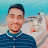Aditya Srivastava says:

Bubble short ka ye bhi shi hai:
#include<iostream>
using namespace std;
int main()
{
int temp=0;
int n;
cin>>n;
int a[n];
for(int i=0;i<n;i++)
{
cin>>a[i];
}
for(int j=0;j<n-1;j++)
{
for(int k=0;k<n-j;k++)
{
if(a[k]>a[k+1])
temp=a[k];
a[k]=a[k+1];
a[k+1]=temp;
}
}
for(int i=0;i<n;i++)
{
cout<<a[i]<<" ";
}
return 0;
}

19.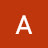Anirudh Rajput says:

20.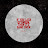2k19-AE-037 MD Wazid Ansari says:

Can anyone tell me if I can go with this code for bubble sort? :")

include<iostream>

using namespace std;

int main()

{

int n,temp;

cout<<"Enter the number of elements : ";

cin>>n;

int arr[n];

for(int i = 0; i<n; ++i)

{

cout<<"Enter the elemenet number "<<i+1<<" : ";

cin>>arr[i];

}

for(int l = 1; l<=n; ++l)

{

for(int j = 0; j<n-1; ++j)

{

if (arr[j]>arr[j+1])

{

temp = arr[j];

arr[j]=arr[j+1];

arr[j+1]=temp;

}

}

}

for(int k = 0; k<n; ++k)

{

cout<<arr[k]<<" ";

}

}

21.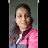Deepa Dixit says:

Apti or DBMS ke liye bhi bana d0 bhaiiyyaa🥺🥺

22.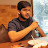Kaustav Saha says:

Urvi didi bulau ya mam😜

23.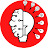Mr. Sukriti Guin says:

Teaching qualities are very well but speed is very high….
Please try to reduce teaching speed…